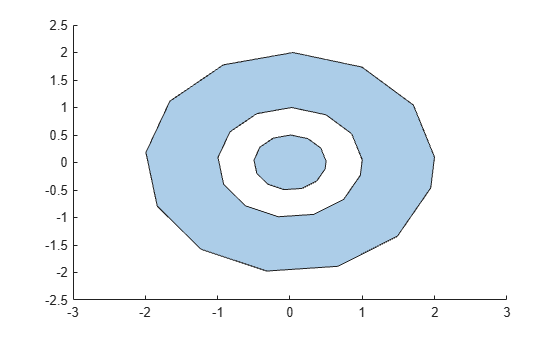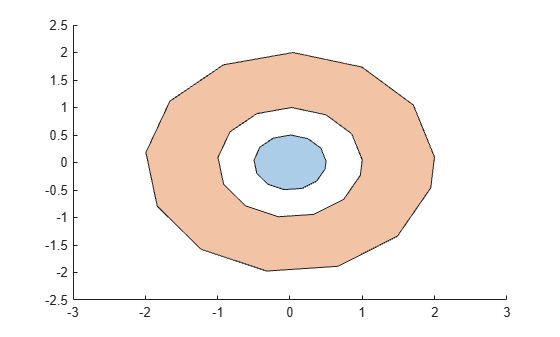# sortregions

Sort `polyshape` regions

## Syntax

``polyout = sortregions(polyin,criterion,direction)``
``polyout = sortregions(polyin,'centroid',direction,'ReferencePoint',point)``

## Description

example

````polyout = sortregions(polyin,criterion,direction)` returns a `polyshape` object whose regions are sorted according to the specified `criterion` and `direction`. For example, `polyout = sortregions(polyin,'area','ascend')` returns a `polyshape` whose solid regions are the same as `polyin`, and listed in ascending order by their area.```
````polyout = sortregions(polyin,'centroid',direction,'ReferencePoint',point)` returns a `polyshape` whose regions are sorted based on the distance of the centroid of each region to a reference point.```

## Examples

collapse all

Identify and access individual regions of a polygon by sorting them first.

Create a polygon with two solid regions and one hole.

```t = 0.05:0.5:2*pi; x1 = cos(t); y1 = sin(t); x2 = 0.5*cos(t); y2 = 0.5*sin(t); x3 = 2*cos(t); y3 = 2*sin(t); polyin = polyshape({x1,x2,x3},{y1,y2,y3})```
```polyin = polyshape with properties: Vertices: [41x2 double] NumRegions: 2 NumHoles: 1 ```
`plot(polyin)`Sort the regions of the polygon in ascending order based on their perimeter. You then can use the `regions` function to create an array of `polyshape` objects `R`, where each element of `R` corresponds to a single region of `polyout`. The elements of `R` are indexed in the same order as the regions of `polyout`, so that you can access and compute with each region based on their index.

```polyout = sortregions(polyin,'perimeter','ascend'); R = regions(polyout); plot(R)````smallregion = area(R(1))`
```smallregion = 0.7541 ```
`bigregion = area(R(2))`
```bigregion = 9.0488 ```

## Input Arguments

collapse all

Input `polyshape`, specified as a scalar, vector, matrix, or multidimensional array.

Data Types: `polyshape`

Sort criterion, specified as one of these values:

• `'area'` — Sort by region area.

• `'perimeter'` — Sort by region perimeter.

• `'numsides'` — Sort by the number of sides of each region.

• `'centroid'` — Sort by the distance from the centroid of each region to the reference point (0,0).

Sort direction, specified as `'ascend'` or `'descend'`.

Reference point, specified as a two-element row vector. The first element is the x-coordinate of the reference point, and the second element is the y-coordinate.